# Wolf method

The Wolf method . In order to calculate a volumetric property, the interaction between an ion and all the rest of the ions up to an infinite distance should be taken into account. However, in practice, summation has to be truncated somewhere. If the truncation is spherical, the sphere considered is not always electrically neutral, as the number of positive and negative ions at a distance no higher than cut radium is not the same. Of course, the charge excess is located in a shell whose thickness equals to the distance between neighbor ions. D. Wolf proposed the approximation that all the charge excess can be considered to be located exactly on the surface of truncation sphere: that is to say to consider the shell infinitely thin. Under this approximation, the potential energy of an ion can be calculated as the summation of the interaction of that ion with the rest of the ions contained in that sphere plus a correction term due to the interaction with the charged surface.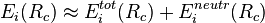$E_{i} (R_{c})\approx E_{i}^{tot}(R_{c}) + E_{i}^{neutr}(R_{c})$

where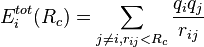$E_{i}^{tot}(R_{c}) = \sum_{j\neq i, r_{ij} < R_{c}}^{} \frac{q_{i}q_{j}}{r_{ij}}$

According to electrostatic rules, the energy of the interaction between an ion with charge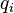$q_{i}$ and a spherical charged surface of radium$R{c}$ centered in the ion$i$ is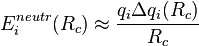$E_{i}^{neutr}(R_{c})\approx \frac{q_{i}\Delta q_{i}(R_{c})}{R_{c}}$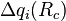$\Delta q_{i}(R_{c})$ is the charge of the sphere of radium$R{c}$ centered in the ion$i$: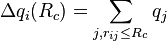$\Delta q_{i}(R_{c})=\sum_{j, r_{ij}\leq R_{c}} q_{j}$

Thus, the energy in the Wolf method is calculated as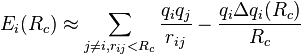$E_{i} (R_{c})\approx \sum_{j\neq i, r_{ij} < R_{c}}^{} \frac{q_{i}q_{j}}{r_{ij}} - \frac{q_{i}\Delta q_{i}(R_{c})}{R_{c}}$

The total potential can be split into two parts: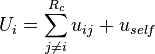$U_{i}=\sum_{j\neq i}^{R_{c}}u_{ij} + u_{self}$

where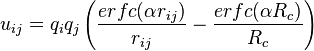$u_{ij}=q_{i}q_{j}\left(\frac{erfc(\alpha r_{ij})}{r_{ij}} - \frac{erfc(\alpha R_{c})}{R_{c}}\right)$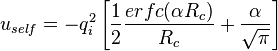$u_{self}= -q_{i}^{2}\left[\frac{1}{2} \frac{erfc(\alpha R_{c})}{R_{c}} + \frac{\alpha}{\sqrt{\pi}} \right]$

In the Wolf method it is suggested that the reciprocal space term of the Ewald Sum may be essentially ignored, provided one compensates the real space contribution by means of a truncation scheme.

## Inhomogeneous systems

It appears to be the case (Ref. 3) that the Wolf method has problems for inhomogeneous systems.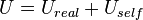$U = U_{real} + U_{self}$